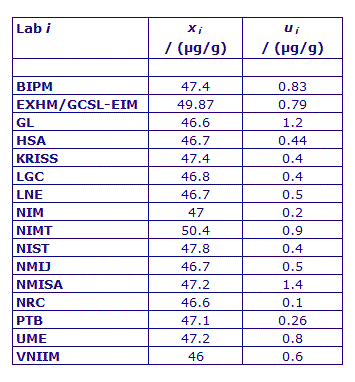Comparison
Comparison close
Results

MEASURAND : Mass fraction of phenylalanine in the CCQM-K78.a sample

Degrees of equivalence : offset Di and expanded uncertainty (k = 2) Ui expressed in µg/g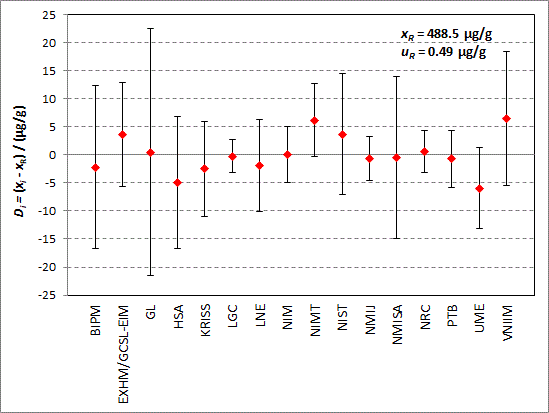MEASURAND : Mass fraction of isoleucine in the CCQM-K78.a sample

Degrees of equivalence : offset Di and expanded uncertainty (k = 2) Ui expressed in µg/g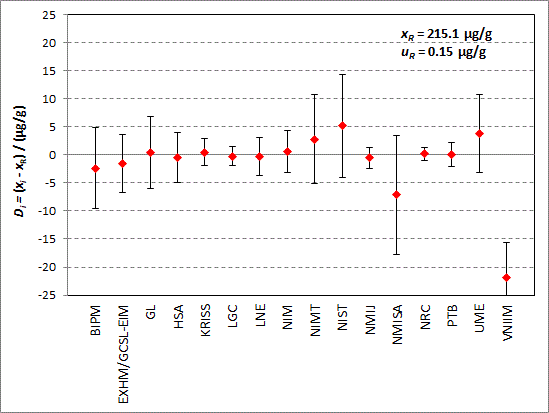MEASURAND : Mass fraction of leucine in the CCQM-K78.a sample

Degrees of equivalence : offset Di and expanded uncertainty (k = 2) Ui expressed in µg/g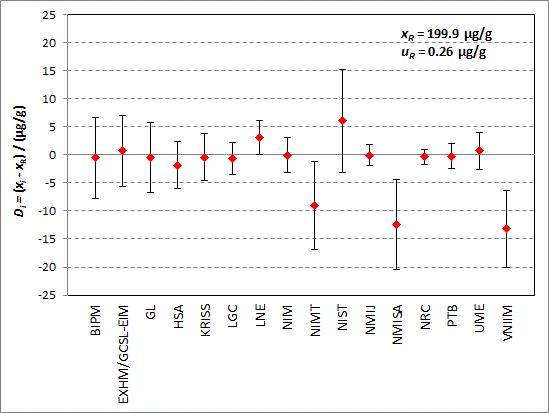MEASURAND : Mass fraction of proline in the CCQM-K78.a sample

Degrees of equivalence : offset Di and expanded uncertainty (k = 2) Ui expressed in µg/g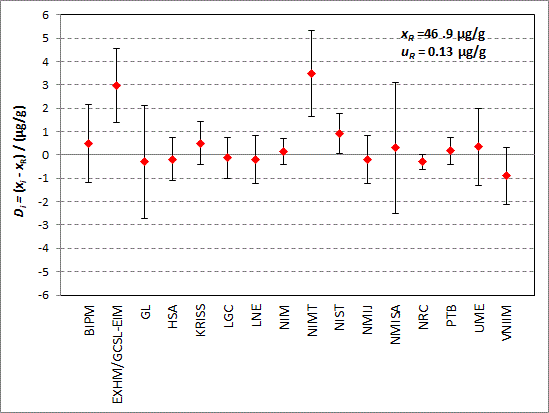Comparison
Comparison close
Results

MEASURAND : Mass fraction of phenylalanine in the CCQM-K78.a sample

Degrees of equivalence : offset Di and expanded uncertainty (k = 2) Ui expressed in µg/g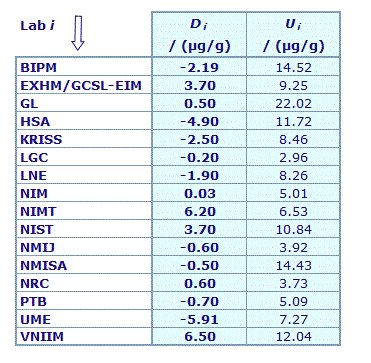Results are presented under A4 printable format in Summary Results (PDF file).

MEASURAND : Mass fraction of isoleucine in the CCQM-K78.a sample

Degrees of equivalence : offset Di and expanded uncertainty (k = 2) Ui expressed in µg/g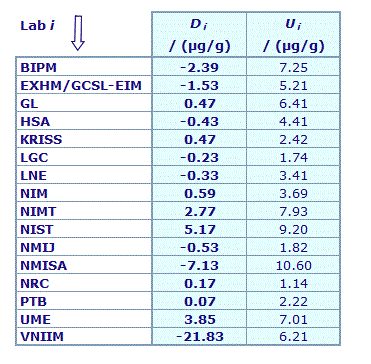Results are presented under A4 printable format in Summary Results (PDF file).

MEASURAND : Mass fraction of leucine in the CCQM-K78.a sample

Degrees of equivalence : offset Di and expanded uncertainty (k = 2) Ui expressed in µg/g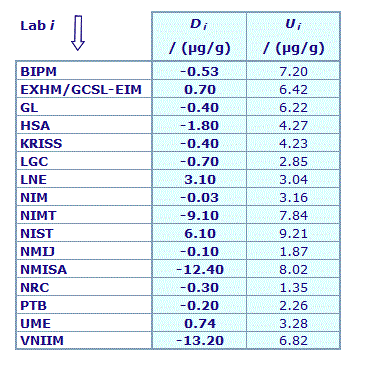Results are presented under A4 printable format in Summary Results (PDF file).

MEASURAND : Mass fraction of proline in the CCQM-K78.a sample

Degrees of equivalence : offset Di and expanded uncertainty (k = 2) Ui expressed in µg/g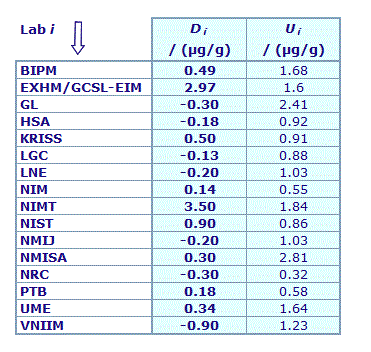Results are presented under A4 printable format in Summary Results (PDF file).

Comparison
Comparison close
CC comparison
 Metrology area, Sub-field Chemistry and Biology, Organics Description Mass fraction assignment of organic analytes in a multi-component aqueous calibration solution Time of measurements 2016 - 2017 Status Approved for equivalence
 Final Reports of the comparisons Measurand Mass fraction in μg/g of Phe in a sample Nominal values in the range 50 μg/g to 500 μg/g Optional: Mass fraction content of Leu, Ile and Pro Transfer device Amber glass ampoules each containing 1 ml of the CCQM-K78.a comparison material, consisting of a gravimetrically prepared solution of amino acids in 0.01 N HCl.
 Comparison type Key Comparison Consultative Committee CCQM (Consultative Committee for Amount of Substance) Conducted by CCQM (Consultative Committee for Amount of Substance) Comments Results published on 14 March 2019 Mass fraction of multicomponent amino acids in dilute HCL solution Pilot institute BIPM Bureau International des Poids et Mesures BIPM - International Organization Contact person Steven Westwood +33 (0) 1 45 07 70 57
First Name Last Name
wwww@ww.www +356719836 Institute 1 Institute 1 Khmelnitskiy
Pilot laboratory
BIPM

Bureau International des Poids et Mesures, BIPM - International Organization, N/A

 EXHM/GCSL-EIM National Laboratory of Chemical Metrology/GCSL - Hellenic Institute of Metrology, Greece, EURAMET
 GL Government Laboratory, Hong Kong, China, APMP
 HSA Health Science Authority, Singapore, APMP
 KRISS Korea Research Institute of Standards and Science, Korea, Republic of, APMP
 LGC Laboratory of the Government Chemist, United Kingdom, EURAMET
 LNE Laboratoire national de métrologie et d'essais, France, EURAMET
 NIM National Institute of Metrology, China, APMP
 NIMT National Institute of Metrology (Thailand), Thailand, APMP
 NIST National Institute of Standards and Technology, United States, SIM
 NMIJ AIST National Metrology Institute of Japan, Japan, APMP
 NMISA National Metrology Institute of South Africa, South Africa, AFRIMETS
 NRC National Research Council, Canada, SIM
 PTB Physikalisch-Technische Bundesanstalt, Germany, EURAMET
 UME TÜBITAK Ulusal Metroloji Enstitüsü, Türkiye, EURAMET
 VNIIM D.I. Mendeleyev Institute for Metrology, Rosstandart, Russian Federation, COOMET
Comparison
Comparison close
Results

MEASURAND : Mass fraction of phenylalanine in the CCQM-K78.a sample

 The key comparison reference value for the mass fraction of phenylalanine xR was obtained from the DerSimonian-Laird variance-weighted mean (DSL-mean) of the participants results excluding outliers. The computation of xR and and its associated uncertainty uR is explained in the page 17 of the Final report. xR = 488.5 µg/g, uR = 0.49 µg/g
 The degree of equivalence of laboratory i with respect to the key comparison reference value is given by a pair of terms: Di = (xi - xR) and Ui, its expanded uncertainty (k = 2), both expressed in µg/g, with Ui = 2(ui2 + uR2)1/2.
 No pair-wise degrees of equivalence are computed for this comparison.

MEASURAND : Mass fraction of isoleucine in the CCQM-K78.a sample

 The key comparison reference value for the mass fraction of phenylalanine xR was obtained from the DerSimonian-Laird variance-weighted mean (DSL-mean) of the participants results excluding outliers. The computation of xR and and its associated uncertainty uR is explained in the page 17 of the Final report. xR = 215.1 µg/g, uR = 0.15 µg/g
 The degree of equivalence of laboratory i with respect to the key comparison reference value is given by a pair of terms: Di = (xi - xR) and Ui, its expanded uncertainty (k = 2), both expressed in µg/g, with Ui = 2(ui2 + uR2)1/2.
 No pair-wise degrees of equivalence are computed for this comparison.

MEASURAND : Mass fraction of leucine in the CCQM-K78.a sample

 The key comparison reference value for the mass fraction of phenylalanine xR was obtained from the DerSimonian-Laird variance-weighted mean (DSL-mean) of the participants results excluding outliers. The computation of xR and and its associated uncertainty uR is explained in the page 17 of the Final report. xR = 199.9 µg/g, uR = 0.26 µg/g
 The degree of equivalence of laboratory i with respect to the key comparison reference value is given by a pair of terms: Di = (xi - xR) and Ui, its expanded uncertainty (k = 2), both expressed in µg/g, with Ui = 2(ui2 + uR2)1/2.
 No pair-wise degrees of equivalence are computed for this comparison.

MEASURAND : Mass fraction of proline in the CCQM-K78.a sample

 The key comparison reference value for the mass fraction of phenylalanine xR was obtained from the DerSimonian-Laird variance-weighted mean (DSL-mean) of the participants results excluding outliers. The computation of xR and and its associated uncertainty uR is explained in the page 17 of the Final report. xR = 46.9 µg/g, uR = 0.13 µg/g
 The degree of equivalence of laboratory i with respect to the key comparison reference value is given by a pair of terms: Di = (xi - xR) and Ui, its expanded uncertainty (k = 2), both expressed in µg/g, with Ui = 2(ui2 + uR2)1/2.
 No pair-wise degrees of equivalence are computed for this comparison.
Comparison
Comparison close
Results

MEASURAND : Mass fraction of phenylalanine in the CCQM-K78.a sample

xi : result of measurement carried out by laboratory i
ui : combined standard uncertainty of xi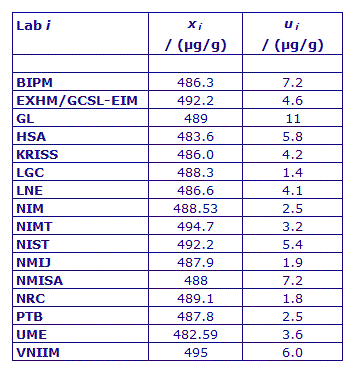MEASURAND : Mass fraction of isoleucine in the CCQM-K78.a sample

xi : result of measurement carried out by laboratory i
ui : combined standard uncertainty of xi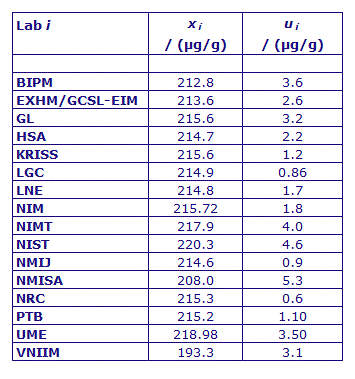MEASURAND : Mass fraction of leucine in the CCQM-K78.a sample

xi : result of measurement carried out by laboratory i
ui : combined standard uncertainty of xi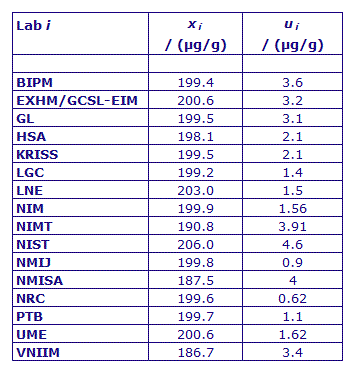MEASURAND : Mass fraction of proline in the CCQM-K78.a sample

xi : result of measurement carried out by laboratory i
ui : combined standard uncertainty of xi